# RS Aggarwal Solutions for Class 8 Maths Chapter 1 - Rational Numbers Exercise 1E

RS Aggarwal Solutions for Class 8 Maths Chapter 1- Rational Numbers, contains solutions to the Exercise 1E questions and can be downloaded from the links provided below. Our team of experts have solved the RS Aggarwal Solutions to ensure that the students get a better understanding of the concepts covered in the textbook and practicing the solutions helps in achieving high marks.

In Exercise 1E of RS Aggarwal Class 8 Maths, we shall study the Division of Rational Numbers and properties of division. In properties, we see Closure property, for every rational number a/b, we have (a/b ÷ 1) = a/b, and for every nonzero rational number a/b, we have (a/b ÷ a/b) = 1.

## Download PDF of RS Aggarwal Solutions for Class 8 Maths Chapter 1- Rational Numbers Exercise 1E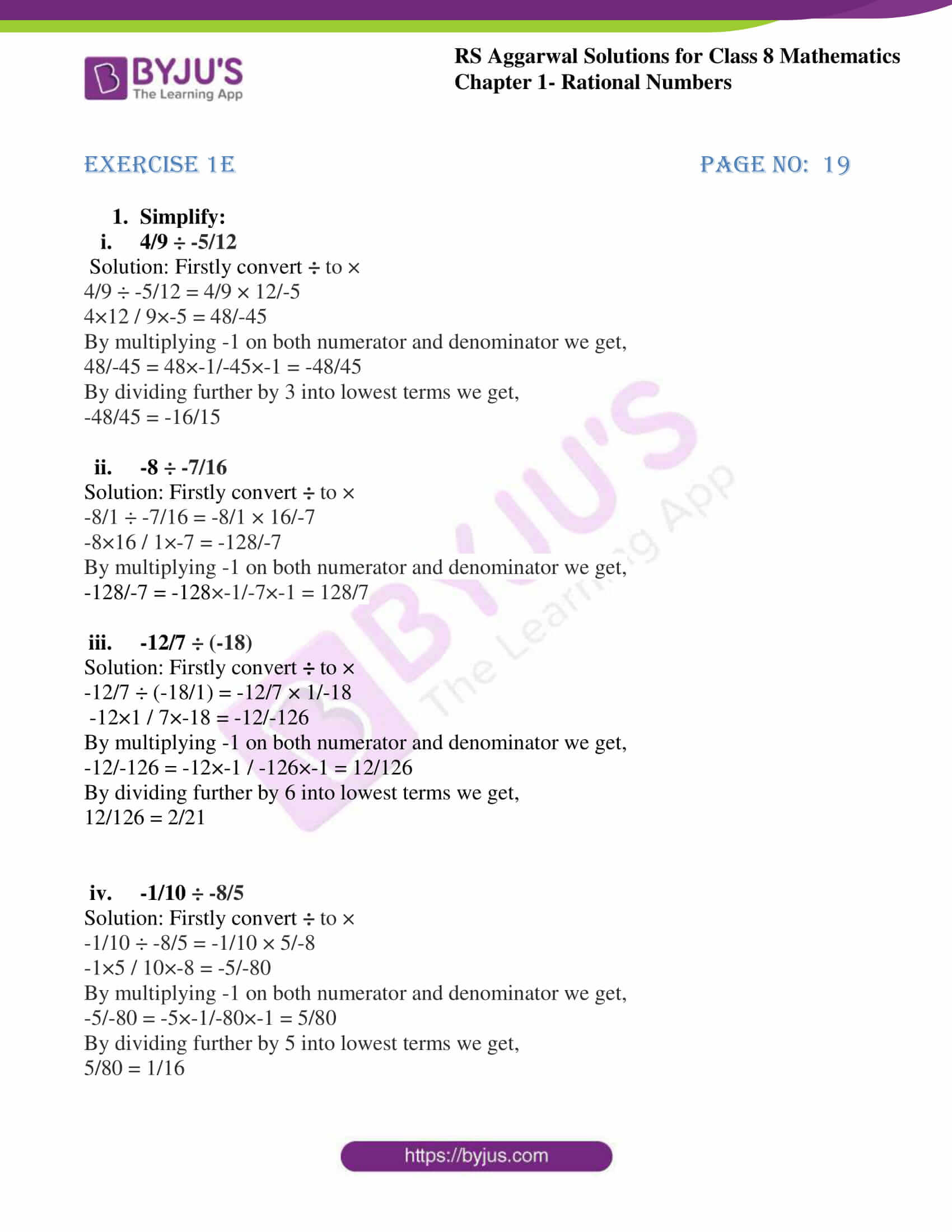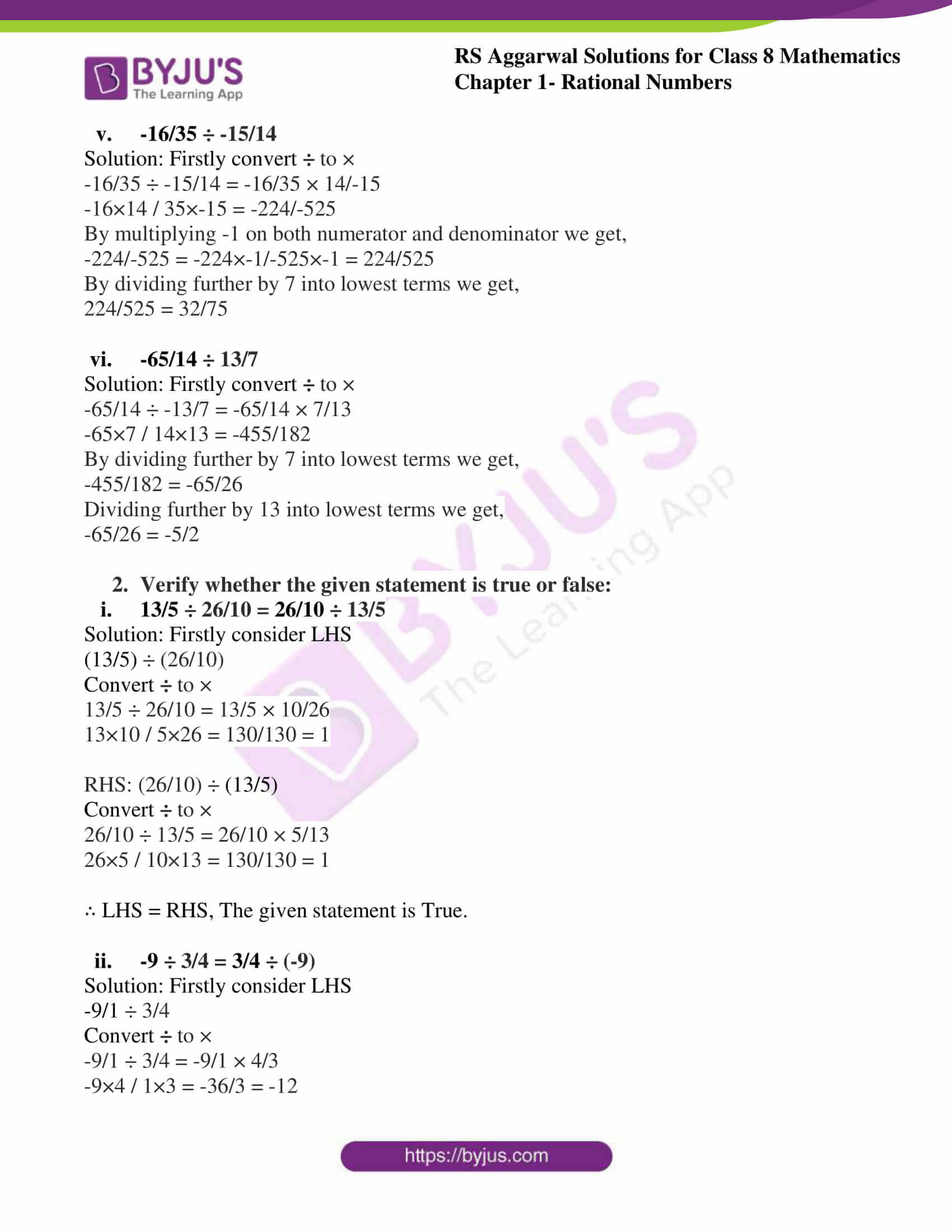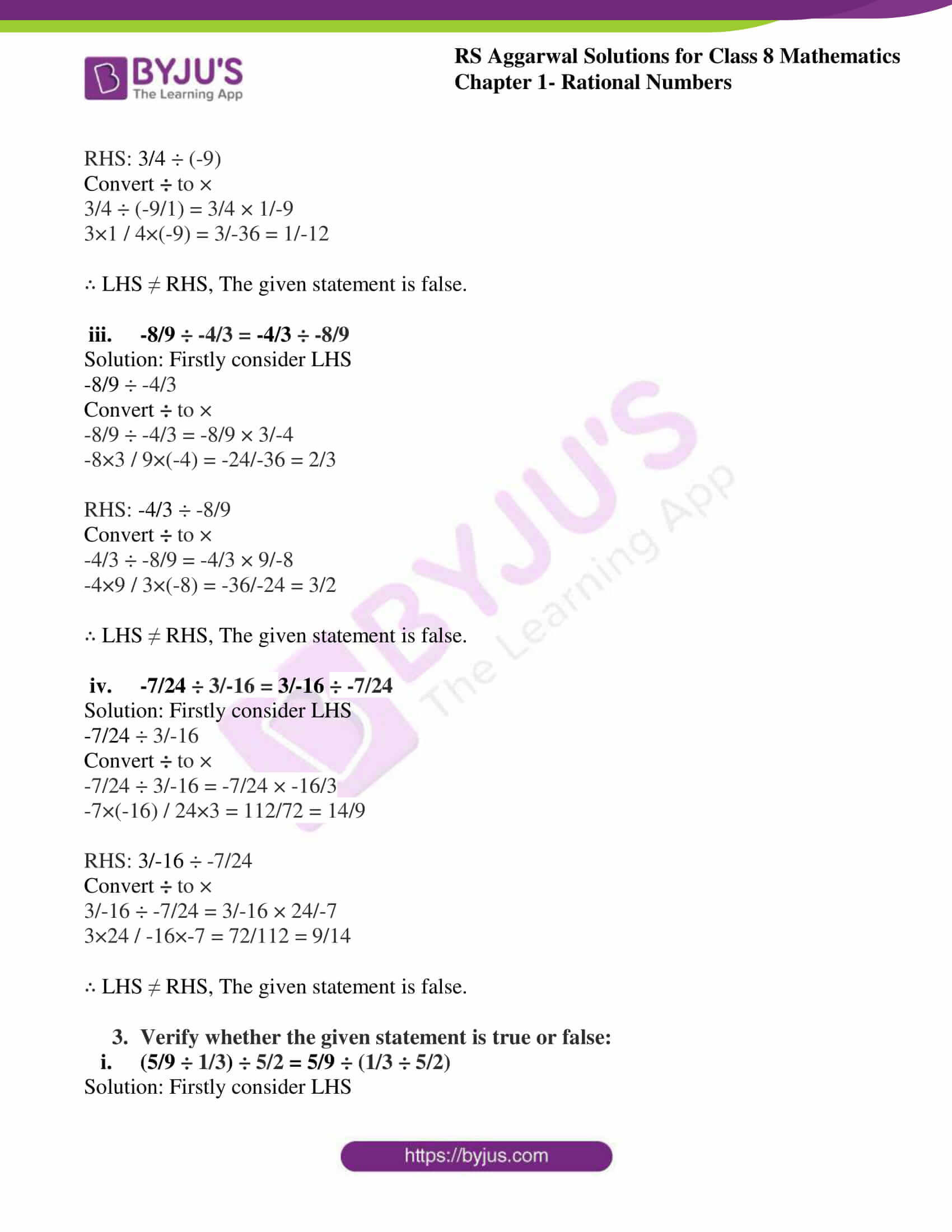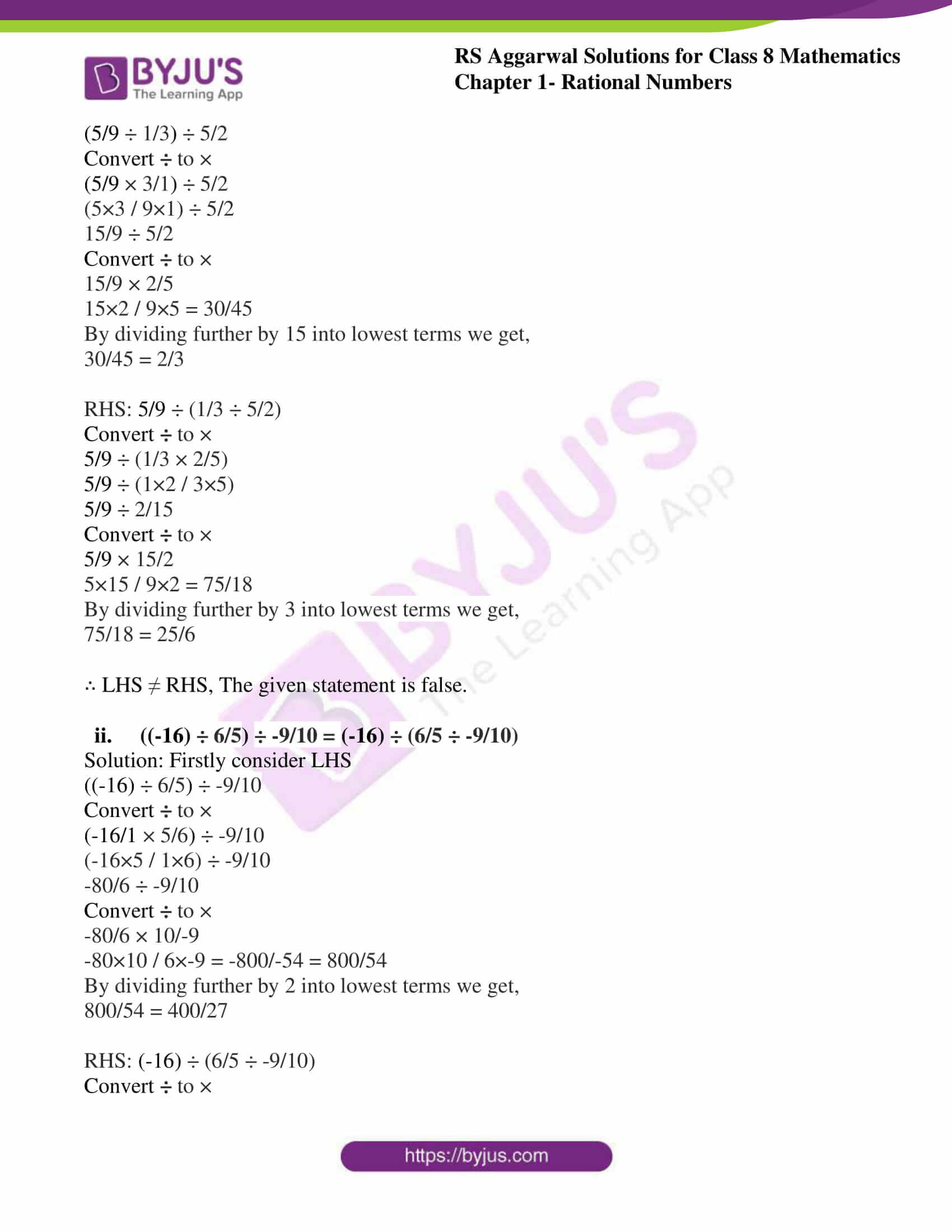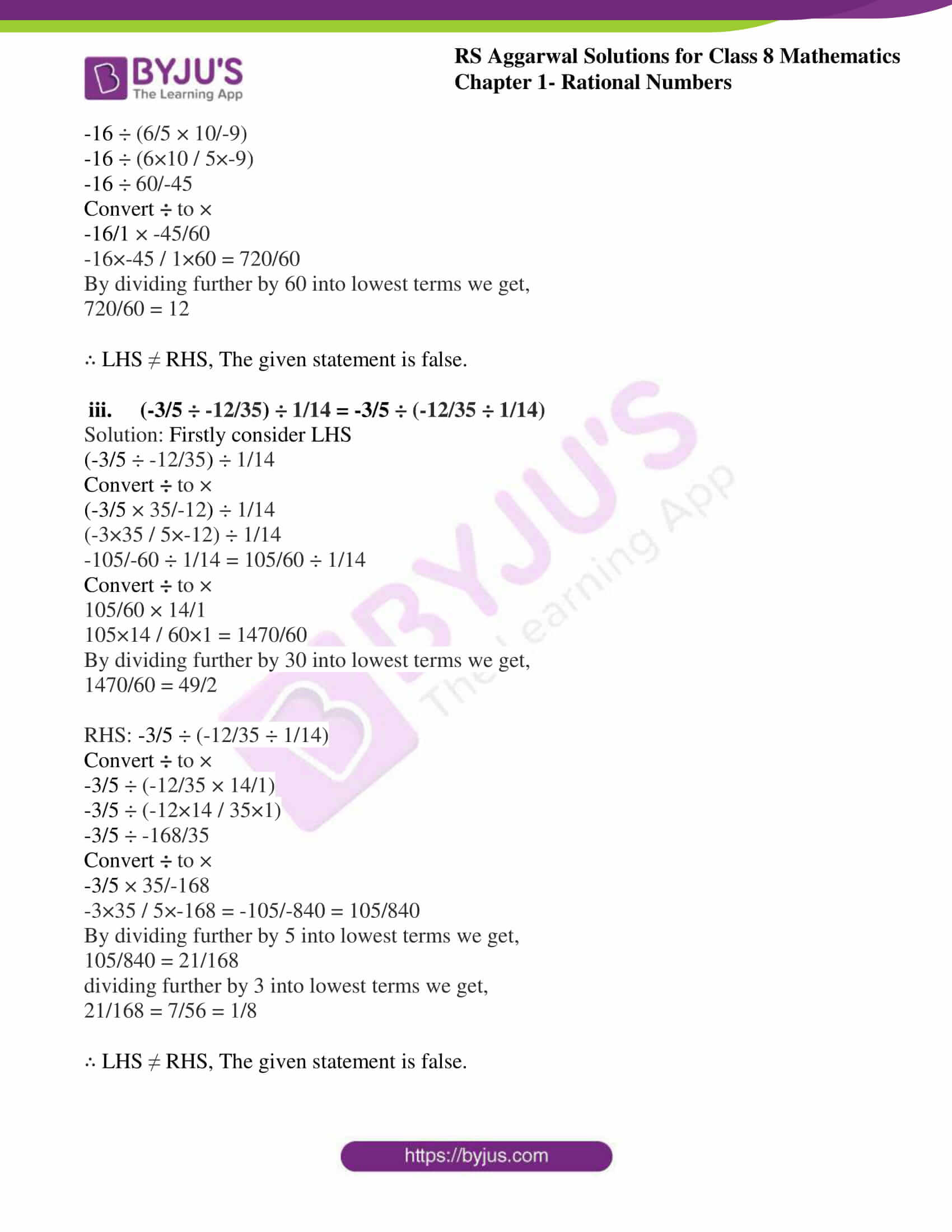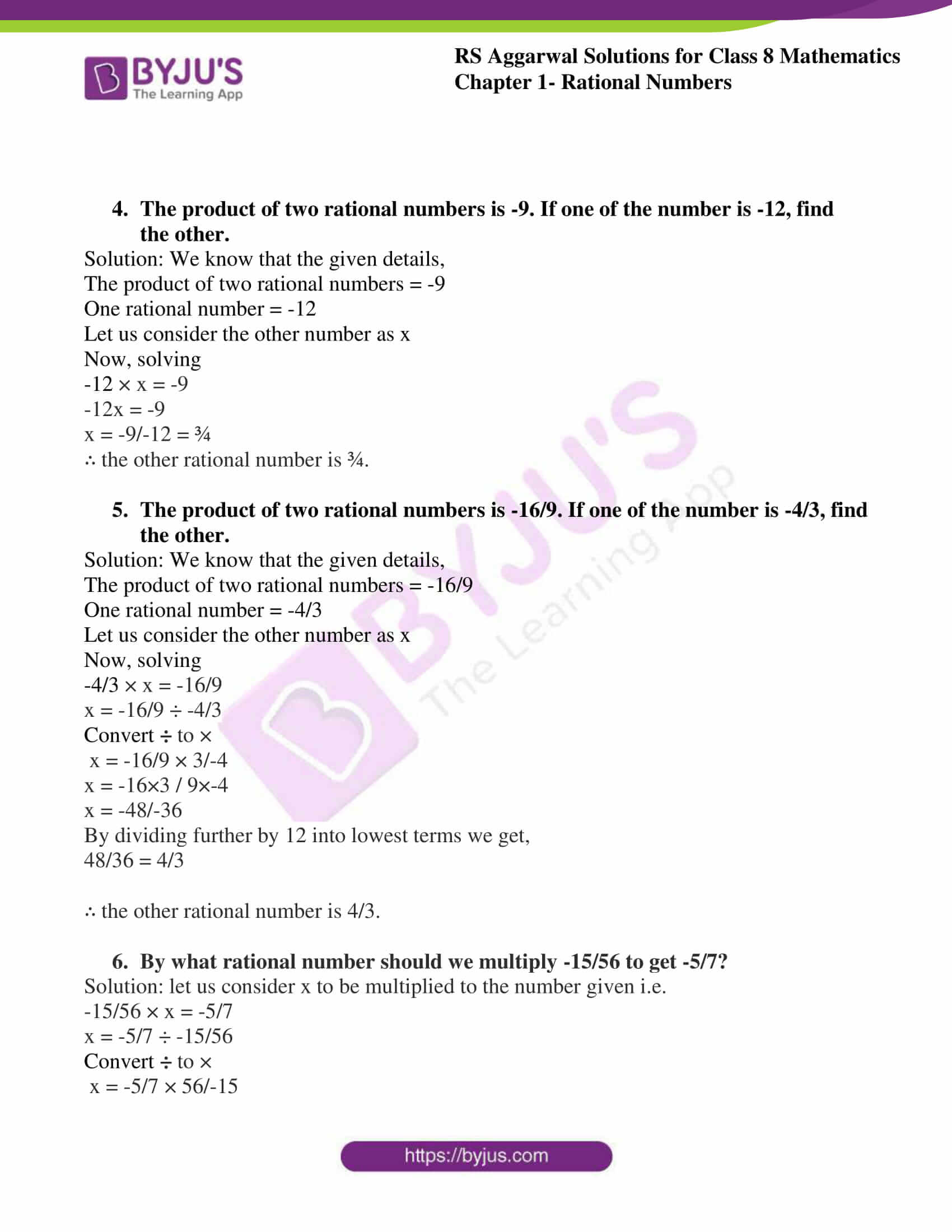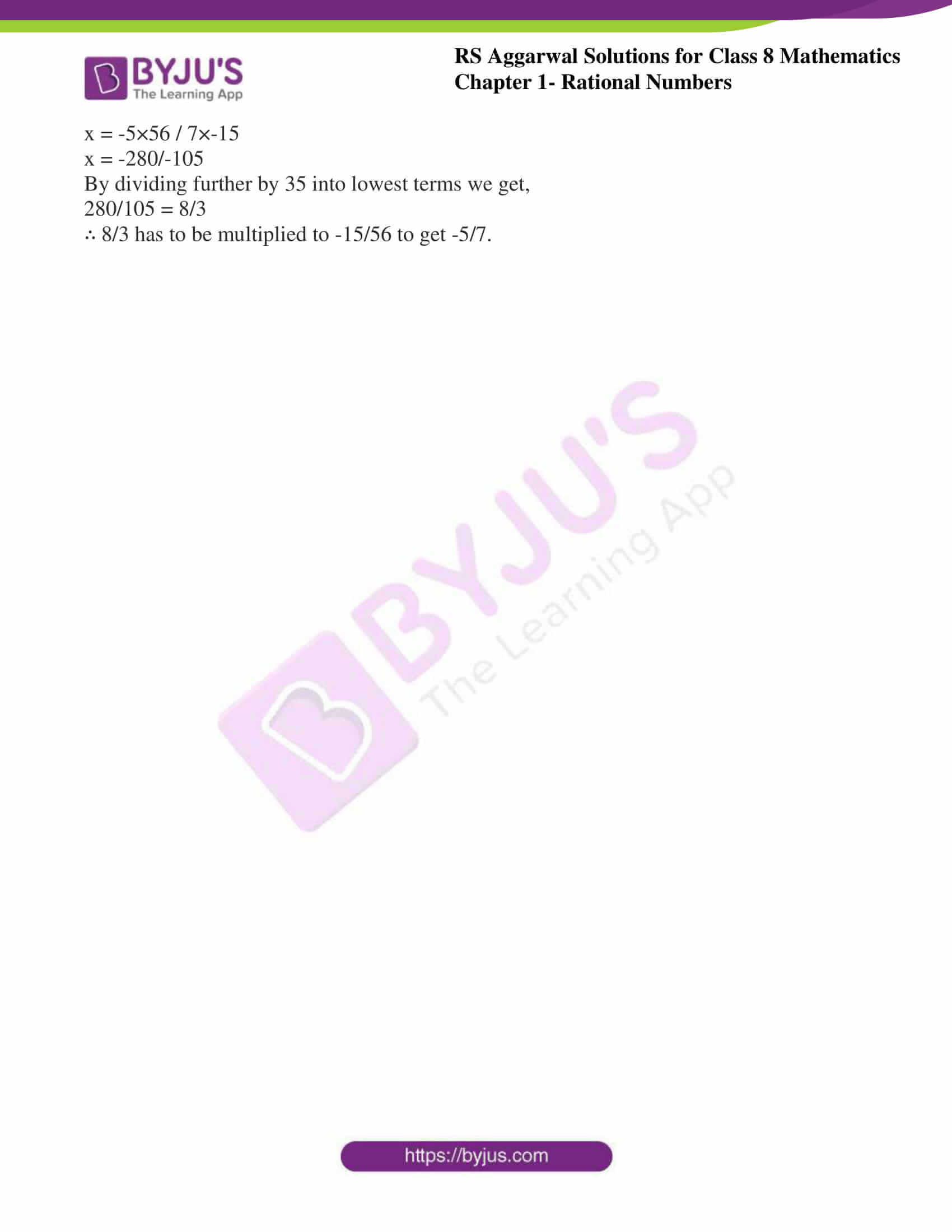### Access Answers to RS Aggarwal Solutions for Class 8 Maths Chapter 1- Rational Numbers Exercise 1E

1. Simplify:

(i) 4/9 ÷ -5/12

Solution:

Firstly convert ÷ to ×

4/9 ÷ -5/12 = 4/9 × 12/-54×12 / 9×-5 = 48/-45

By multiplying -1 on both numerator and denominator we get,

48/-45 = 48×-1/-45×-1 = -48/45

By dividing further by 3 into lowest terms we get,

-48/45 = -16/15

(ii) -8 ÷ -7/16

Solution:

Firstly convert ÷ to ×

-8/1 ÷ -7/16 = -8/1 × 16/-7-8×16 / 1×-7 = -128/-7

By multiplying -1 on both numerator and denominator we get,

-128/-7 = -128×-1/-7×-1 = 128/7

(iii) -12/7 ÷ (-18)

Solution:

Firstly convert ÷ to ×

-12/7 ÷ (-18/1) = -12/7 × 1/-18-12×1 / 7×-18 = -12/-126

By multiplying -1 on both numerator and denominator we get,

-12/-126 = -12×-1 / -126×-1 = 12/126

By dividing further by 6 into lowest terms we get,

12/126 = 2/21

(iv) -1/10 ÷ -8/5

Solution:

Firstly convert ÷ to ×

-1/10 ÷ -8/5 = -1/10 × 5/-8-1×5 / 10×-8 = -5/-80

By multiplying -1 on both numerator and denominator we get,

-5/-80 = -5×-1/-80×-1 = 5/80

By dividing further by 5 into lowest terms we get,

5/80 = 1/16

(v) -16/35 ÷ -15/14

Solution:

Firstly convert ÷ to ×

-16/35 ÷ -15/14 = -16/35 × 14/-15-16×14 / 35×-15 = -224/-525

By multiplying -1 on both numerator and denominator we get,

-224/-525 = -224×-1/-525×-1 = 224/525

By dividing further by 7 into lowest terms we get,

224/525 = 32/75

(vi) -65/14 ÷ 13/7

Solution:

Firstly convert ÷ to ×

-65/14 ÷ -13/7 = -65/14 × 7/13-65×7 / 14×13 = -455/182

By dividing further by 7 into lowest terms we get,

-455/182 = -65/26

Dividing further by 13 into lowest terms we get,

-65/26 = -5/2

2. Verify whether the given statement is true or false:

(i) 13/5 ÷ 26/10 = 26/10 ÷ 13/5

Solution:

Firstly consider LHS

(13/5) ÷ (26/10)

Convert ÷ to ×

13/5 ÷ 26/10 = 13/5 × 10/26

13×10 / 5×26 = 130/130 = 1

RHS: (26/10) ÷ (13/5)

Convert ÷ to ×

26/10 ÷ 13/5 = 26/10 × 5/13

26×5 / 10×13 = 130/130 = 1

∴ LHS = RHS, The given statement is True.

(ii) -9 ÷ 3/4 = 3/4 ÷ (-9)

Solution:

Firstly consider LHS

-9/1 ÷ 3/4

Convert ÷ to ×

-9/1 ÷ 3/4 = -9/1 × 4/3

-9×4 / 1×3 = -36/3 = -12

RHS: 3/4 ÷ (-9)

Convert ÷ to ×

3/4 ÷ (-9/1) = 3/4 × 1/-9

3×1 / 4×(-9) = 3/-36 = 1/-12

∴ LHS ≠ RHS, The given statement is false.

(iii) -8/9 ÷ -4/3 = -4/3 ÷ -8/9

Solution:

Firstly consider LHS

-8/9 ÷ -4/3

Convert ÷ to ×

-8/9 ÷ -4/3 = -8/9 × 3/-4

-8×3 / 9×(-4) = -24/-36 = 2/3

RHS: -4/3 ÷ -8/9

Convert ÷ to ×

-4/3 ÷ -8/9 = -4/3 × 9/-8

-4×9 / 3×(-8) = -36/-24 = 3/2

∴ LHS ≠ RHS, The given statement is false.

(iv) -7/24 ÷ 3/-16 = 3/-16 ÷ -7/24

Solution:

Firstly consider LHS

-7/24 ÷ 3/-16

Convert ÷ to ×

-7/24 ÷ 3/-16 = -7/24 × -16/3

-7×(-16) / 24×3 = 112/72 = 14/9

RHS: 3/-16 ÷ -7/24

Convert ÷ to ×

3/-16 ÷ -7/24 = 3/-16 × 24/-7

3×24 / -16×-7 = 72/112 = 9/14

∴ LHS ≠ RHS, The given statement is false.

3. Verify whether the given statement is true or false:

(i) (5/9 ÷ 1/3) ÷ 5/2 = 5/9 ÷ (1/3 ÷ 5/2)

Solution:

Firstly consider LHS

(5/9 ÷ 1/3) ÷ 5/2

Convert ÷ to ×

(5/9 × 3/1) ÷ 5/2

(5×3 / 9×1) ÷ 5/2

15/9 ÷ 5/2

Convert ÷ to ×

15/9 × 2/5

15×2 / 9×5 = 30/45

By dividing further by 15 into lowest terms we get,

30/45 = 2/3

RHS: 5/9 ÷ (1/3 ÷ 5/2)

Convert ÷ to ×

5/9 ÷ (1/3 × 2/5)

5/9 ÷ (1×2 / 3×5)

5/9 ÷ 2/15

Convert ÷ to ×

5/9 × 15/2

5×15 / 9×2 = 75/18

By dividing further by 3 into lowest terms we get,

75/18 = 25/6

∴ LHS ≠ RHS, The given statement is false.

(ii) ((-16) ÷ 6/5) ÷ -9/10 = (-16) ÷ (6/5 ÷ -9/10)

Solution:

Firstly consider LHS

((-16) ÷ 6/5) ÷ -9/10

Convert ÷ to ×

(-16/1 × 5/6) ÷ -9/10

(-16×5 / 1×6) ÷ -9/10

-80/6 ÷ -9/10

Convert ÷ to ×

-80/6 × 10/-9

-80×10 / 6×-9 = -800/-54 = 800/54

By dividing further by 2 into lowest terms we get,

800/54 = 400/27

RHS: (-16) ÷ (6/5 ÷ -9/10)

Convert ÷ to ×

-16 ÷ (6/5 × 10/-9)

-16 ÷ (6×10 / 5×-9)

-16 ÷ 60/-45

Convert ÷ to ×

-16/1 × -45/60

-16×-45 / 1×60 = 720/60

By dividing further by 60 into lowest terms we get,

720/60 = 12

∴ LHS ≠ RHS, The given statement is false.

(iii) (-3/5 ÷ -12/35) ÷ 1/14 = -3/5 ÷ (-12/35 ÷ 1/14)

Solution:

Firstly consider LHS

(-3/5 ÷ -12/35) ÷ 1/14

Convert ÷ to ×

(-3/5 × 35/-12) ÷ 1/14

(-3×35 / 5×-12) ÷ 1/14

-105/-60 ÷ 1/14 = 105/60 ÷ 1/14

Convert ÷ to ×

105/60 × 14/1

105×14 / 60×1 = 1470/60

By dividing further by 30 into lowest terms we get,

1470/60 = 49/2

RHS: -3/5 ÷ (-12/35 ÷ 1/14)

Convert ÷ to ×

-3/5 ÷ (-12/35 × 14/1)

-3/5 ÷ (-12×14 / 35×1)

-3/5 ÷ -168/35

Convert ÷ to ×

-3/5 × 35/-168

-3×35 / 5×-168 = -105/-840 = 105/840

By dividing further by 5 into lowest terms we get,

105/840 = 21/168

dividing further by 3 into lowest terms we get,

21/168 = 7/56 = 1/8

∴ LHS ≠ RHS, The given statement is false.

4. The product of two rational numbers is -9. If one of the number is -12, find the other.

Solution: We know that the given details,

The product of two rational numbers = -9

One rational number = -12

Let us consider the other number as x

Now, solving

-12 × x = -9

-12x = -9

x = -9/-12 = ¾

∴ the other rational number is ¾.

5. The product of two rational numbers is -16/9. If one of the number is -4/3, find the other.

Solution: We know that the given details,

The product of two rational numbers = -16/9

One rational number = -4/3

Let us consider the other number as x

Now, solving

-4/3 × x = -16/9

x = -16/9 ÷ -4/3

Convert ÷ to ×

x = -16/9 × 3/-4

x = -16×3 / 9×-4

x = -48/-36

By dividing further by 12 into lowest terms we get,

48/36 = 4/3

∴ the other rational number is 4/3.

6. By what rational number should we multiply -15/56 to get -5/7?

Solution: let us consider x to be multiplied to the number given i.e.

-15/56 × x = -5/7

x = -5/7 ÷ -15/56

Convert ÷ to ×

x = -5/7 × 56/-15

x = -5×56 / 7×-15

x = -280/-105

By dividing further by 35 into lowest terms we get,

280/105 = 8/3

∴ 8/3 has to be multiplied to -15/56 to get -5/7.

### Access other Exercises of RS Aggarwal Solutions for Class 8 Maths Chapter 1- Rational Numbers

Exercise 1A Solutions 10 Questions

Exercise 1B Solutions 3 Questions

Exercise 1C Solutions 14 Questions

Exercise 1D Solutions 9 Questions

Exercise 1F Solutions 8 Questions

Exercise 1G Solutions 20 Questions

Exercise 1H Solutions 23 Questions

### RS Aggarwal Solutions for Class 8 Maths Chapter 1- Rational Numbers Exercise 1E

Exercise 1E of RS Aggarwal Class 8 Chapter 1, Rational Numbers contains the advance concepts related to Rational Numbers. This exercise mainly deals with the Division of rational numbers and the properties of division.

The RS Aggarwal Solutions can help the students practice and learn the fundamentals as it provides all the answers to the questions from the RS Aggarwal textbook. Students should practice RS Aggarwal Solutions without fail to understand how the concepts are solved. Practicing as many times as possible helps in time management skills and also increases the confidence level to attain high marks.